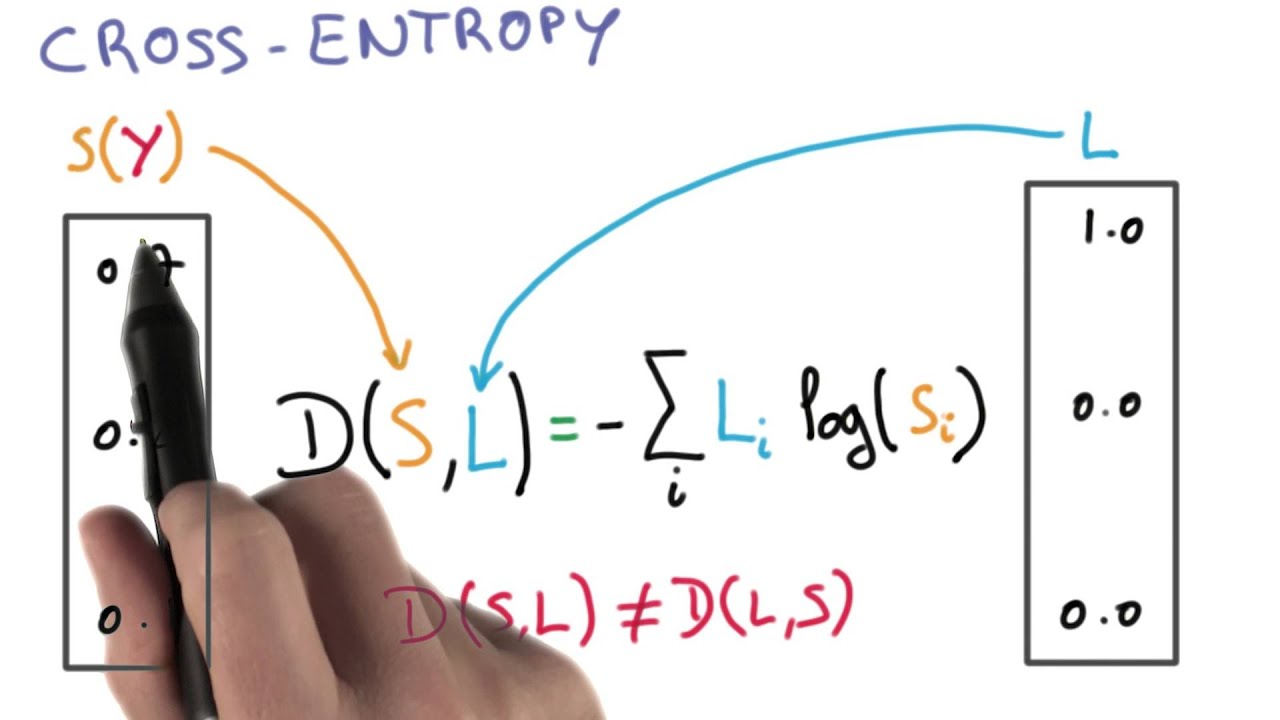/  Deep Learning Interview questions and answers   /  Explain difference between sparse categorical cross entropy and categorical entropy?## Explain difference between sparse categorical cross entropy and categorical entropy?

Ans: For both sparse categorical cross entropy and categorical cross entropy have same loss functions but only difference is the format.

J(w)=−1N∑i=1N[yilog(y^i)+(1−yi)log(1−y^i)]

Where

w refers to the model parameters, e.g. weights of the neural network

yi is the true label

yi^ is the predicted label

If your Yi’s are one-hot encoded, use categorical cross entropy. But if your Yi’s are integers, use sparse cross entropy. The usage entirely depends on loading the dataset. Advantage of using sparse categorical cross entropy is, it saves time in memory as well as speed up the computation process.